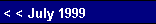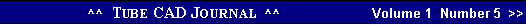Tube Shunt Regulators
 how few parts are required. Still this regulator is only adequate at maintaining a constant output voltage. A decrease in current draw of 1 mA at its output that would  in the absence of the triode cause a 1 volt increase in output  voltage. But with the triode in place, the rp is effectively in parallel with the 1k resistor, which yields an equivalent resistance of only 285 ohms and 1 mA multiplied against this value results only a .285 volt increase in plate voltage. True, this is only a modest improvement in regulation, but the triode has that third element that greatly increases the regulation.   The amplification factor or mu of a triode is the measure
 Shunt regulators offer many sonic advantages over series regulators; they usually find a shorter and cleaner path to the ground reference, and they absorb noise rather than transmit it, but at the cost of decreased efficiency. Inefficiency, however, is something with which tube audio designers have learned to accept and some believe to be virtue: "the less efficient, the better the sound."       The principle of operation of a shunt regulator in AC terms is simple enough: the noise signal on the power supply rail is compared to ground, then the current through the shunt regulator is varied in response to that signal, and then this current develops a varying voltage across a resistor or choke that cancels this same signal. In DC terms: the power supply rail voltage is compared to a voltage reference and the idle current through the shunt regulator is varied to bring the power supply voltage in line with the reference.    Like the series regulator, the shunt regulator needs three elements to work: a shunting device with a variable conductance, a voltage reference that is AC bypassed to ground, and a feedback mechanism to control the flow of current through the shunt regulator. Amazingly enough, the triode comprises all three elements at once. The triode's current can be varied by varying grid-to-cathode voltage, which is why the triode is called a "valve" in England. The same grid-to-cathode voltage creates a soft voltage reference, as the triode's plate will buck or fight any change in plate voltage. This occurs because a triode, unlike a pentode or a transistor or MOSFET, exhibits plate resistance (rp). For example, a 4k resistor attached to a power supply of 400 volts will experience a current flow of 100 mA. Moving the power supply voltage up and down will increase and decrease the current flow through the resistor, as         DI = DV / R.If we replace the resistor with a triode with the correct grid-to-cathode voltage, the same current would flow as did with the resistor. But here is the important difference: if we move the power supply voltage up and down, current will increase and decrease much more severely than it did through the resistor, because the rp of the triode might only 400 ohms,          DI = DV / rp.Before going on to the third element, we can see that a triode gives us all we need to make a simple shunt regulator.   These two examples of a simple shunt regulator show just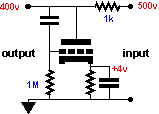Shunt regulator using a triode with feedback from the plate to the cathode to lower the output impedance.
 of the degree that the grid is more effective than the plate in controlling the flow of current through the tube. Here is our missing feedback mechanism to control the flow of current through the shunt regulator. By delivering the change in output voltage of the regulator to the grid of the triode, the amount of regulation increases by the mu of the triode. For example if the mu of the triode is 35, then the 1 mA current decrease would only result in a .0011 volt, as the output impedance of the regulator is about 11 ohms:       Zo = (Ra||rp) /Gainbut as          Gain = µRa / (Ra + rp)and            Ra||rp = Ra•rp / (Ra + rp)thus      Zo = [Ra•rp / (Ra + rp)] / [µRa / (Ra + rp)]      Zo = rp / µ    or   Zo = 1 / Gm.In this case      Zo = 400 / 35.    So we see that what really matters is Gm (transconductance), as the greater the Gm, the lower the output impedance. One triode might have a mu of 10 and an rp of 1000; and another, a mu of 100 and an rp of 10000. But each triode would still have an equal Gm of 10 mA/v or as it is often expressed in micromhos, 10,000 µmhos, as       Gm = µ / rp. Yet if given this choice of triodes, the better sonic choice might be the lower mu tube, as it is easier to make a very linear low mu triode than a very linear high mu triode. ( The 6BX7 and the 6BL7 are identical triodes save for the distance of the grid to the cathode. This distance is greater in the 6BX7, which lowers its mu and probably explains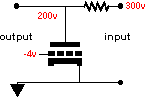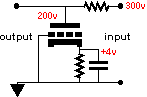pg. 12